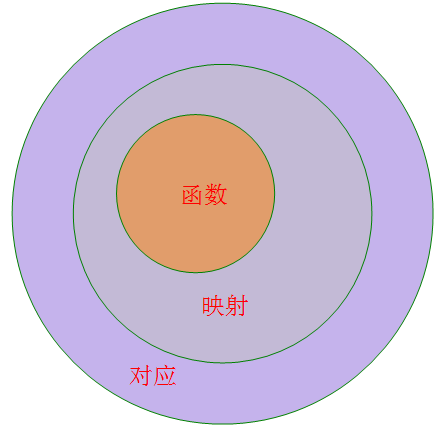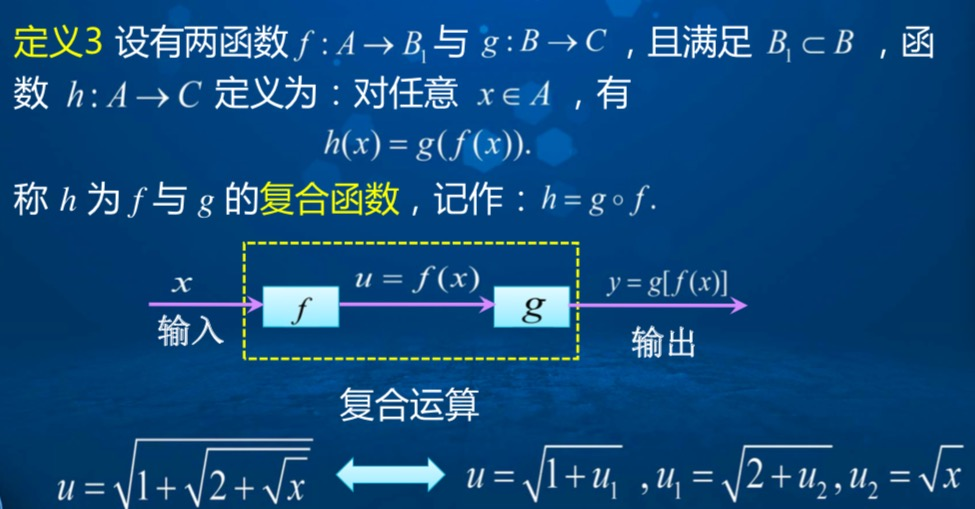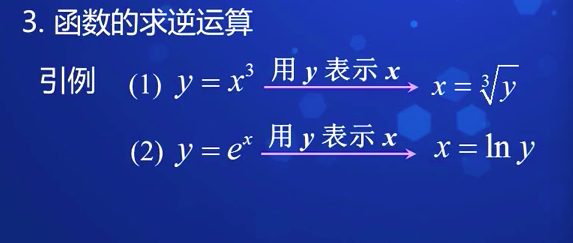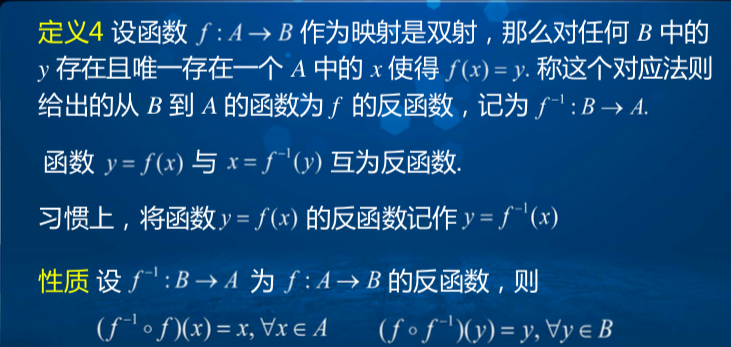• 使用MATLAB过程中，我们难免会遇到函数调用函数的情况，外面的主函数中的变量我们可以工作区看到，他们的值的变化我们可以清楚的知道，但是主程序中调用的一些函数的变量的值我们却很难知道，此时，我们可以采取...
matlab中如何知道主函数调用的其他函数中的变量值
在使用MATLAB过程中，我们难免会遇到函数调用函数的情况，外面的主函数中的变量我们可以在工作区看到，他们的值的变化我们可以清楚的知道，但是主程序中调用的一些函数的变量的值我们却很难知道，此时，我们可以采取如下方法：
（1）将主程序中函数末尾的分号去掉，再次运行程序，在命令行窗口我们就可以看到函数中自变量的值；
然而，被调用函数的程序中的一些变量、常量的值我们如何知道呢？
（2）在被调用函数的某处直接加入一段程序即可，这段程序就是你这个想知道的变量的名字，不加分号，回到主程序，运行，即可在***命令行窗口***看到这个变量的变化的值。


展开全文matlab
• 然后针对降水量为相依随机变量的特点 ,采取以规范化的各阶相关系数为权重 ,用加权的马尔可夫链模型来预测未来降水的丰枯变化状况 ;最后以山西省水文站近 5 0年的降水资料为实例对该方法进行了具体的应用 ,获得了...
• 三、函数定义定义 设 与 是过程中的两个变量,如果当变量 在变化范围 中任取一个数值时,变量 按照一定对应规则,总有确定数值和它相对应,则称变量 为变量 函数,记作 ,其中 称为自变量, 称为因变量, 称为...2019-03-11函数是什么？三、函数的定义定义 设  与  是某一过程中的两个变量,如果当变量  在变化范围  中任取一个数值时,变量  按照一定的对应规则,总有确定的数值和它相对应,则称变量  为变量  的函数,记作  ,其中  称为自变量,  称为因变量,  称为对应法则。函数  当  时的函数值，记作  或  。自变量  的变化范围  称为函数的定义域, 函数  取值的全体称为函数的值域。如，圆的面积  与它的半径  之间的关系由公式  给定, 面积  与它的半径  都是变量,当半径  在区间  内任意取定一个数值时,由  就确定一个相应的圆面积  的数值,因此面积  是它的半径  的函数。又...全部三、函数的定义定义 设  与  是某一过程中的两个变量,如果当变量  在变化范围  中任取一个数值时,变量  按照一定的对应规则,总有确定的数值和它相对应,则称变量  为变量  的函数,记作  ,其中  称为自变量,  称为因变量,  称为对应法则。函数  当  时的函数值，记作  或  。自变量  的变化范围  称为函数的定义域, 函数  取值的全体称为函数的值域。如，圆的面积  与它的半径  之间的关系由公式  给定, 面积  与它的半径  都是变量,当半径  在区间  内任意取定一个数值时,由  就确定一个相应的圆面积  的数值,因此面积  是它的半径  的函数。又如，  中  都是变量,当变量  在区间  内任意取定一个数值时,变量  有两个确定的值与  对应, 因此变量  是变量  的函数。关于函数的定义做以下说明:1。符号“  ”的意义符号“  ”表示自变量  与函数  的某种对应规则。例如  ,它的对应法则“  ”就是自变量的平方乘以2减去自变量的3倍加上1,不妨简化为  。如，当  时,对应的函数值为  ;同样当  时,对应的函数值为  。2。单值函数和多值函数如果函数  对定义域内任一自变量的值都只有一个确定的值与之对应,这个函数就称为单值函数,如果有两个或更多的值与之对应,就称为多值函数。如举例中的  为单值函数,  为多值函数。以后谈到的函数如无特别说明都是指单值函数。3。确定函数的两个要素——定义域、对应法则。所谓两个函数相同，是指它们的定义域和对应法则均相同。如， 函数   与   不是相同的两个函数。事实上   的定义域为:  ,的定义域为:  ,由于两个函数的定义域不同,所以两个函数不相同。又如，函数   与   是相同的函数。事实上，  的定义域为:  ,对应法则为:  ,的定义域为:  ,对应法则为:  。由于两个函数的定义域和对应法则都相同,只是自变量和因变量所选字母不同,所以两个函数相同。在求函数定义域时,常常遇到下面几种情况:(1) 若函数  是多项式,则定义域为一切实数;(2) 若函数  是分式函数,则要求分式的分母的表达式不为零。(3) 若函数  是偶次根式函数,则要求偶次根号下的表达式非负。(4) 若函数  是对数函数, 则要求对数中的真数表达式大于零。(5) 若函数  是由几部分代数式组成,则定义域是使这几部分代数式都有意义的  值的全体。例1         求  的定义域。分析  由对数中的真数表达式大于零知，         ,由根式中的偶次根号下的表达式非负知，   ,由分式分母的表达式不为零知，           ,联立求解即可。解   应使    ， 即    。   所以   ， 故定义域为:  。  。收起
展开全文• 传统定义：设在某变化过程中有两个变量$$x$$、$$y$$，如果对于$$x$$某一范围内每一个确定值，$$y$$都有唯一确定值与它对应，那么就称$$y$$是$$x$$函数，$$x$$叫做自变量。我们将自变量$$x$$取值集合叫做...
一、关于函数的概念：
函数的概念有两个，其一为初中的定义，称为传统定义，其二为高中的定义，称为近代定义。
传统定义：设在某变化过程中有两个变量$$x$$、$$y$$，如果对于$$x$$在某一范围内的每一个确定的值，$$y$$都有唯一确定的值与它对应，那么就称$$y$$是$$x$$的函数，$$x$$叫做自变量。我们将自变量$$x$$取值的集合叫做函数的定义域，和自变量$$x$$对应的$$y$$的值叫做函数值，函数值的集合叫做函数的值域。
近代定义：设$$A$$，$$B$$都是非空的数集，$$f：x→y$$是从$$A$$到$$B$$的一个对应法则，那么从$$A$$到$$B$$的映射$$f：A→B$$就叫做函数，记作$$y=f(x)$$，其中$$x∈A$$，$$y∈B$$，原象集合$$A$$叫做函数$$f(x)$$的定义域，象集合$$C$$叫做函数$$f(x)$$的值域，显然有$$C\subseteq B$$。
对函数概念的理解
函数的两个定义本质是一致的，只是叙述概念的出发点不同，传统定义是从运动变化的观点出发，而近代定义是从集合、映射的观点出发。这样，就不难得知函数实质是从非空数集A到非空数集B的一个特殊的映射。
二、基于对应基础的函数概念的理解（近代定义）
（1）首先需要先搞清楚对应的概念，
关于对应的概念，我们基于蜜蜂采蜜的生活常识来理解，可以一只蜜蜂采一朵花（称为“一对一”的对应），
可以一只蜜蜂采多朵花（称为“一对多”的对应），还可以多只蜜蜂采一朵花（称为“多对一”的对应）
即对应有一对一，一对多和多对一三种对应关系。（2）映射
能够称为映射的对应只有一对一和多对一两种，其中一对多不能称为映射，
映射$$f：A\rightarrow B$$和映射$$f：B\rightarrow A$$是不一样的。
集合$$A，B$$不一定是数集，可以是图形集，式集，点集，向量集等，
（3）函数
非空数集$$A$$到非空数集$$B$$的映射$$f：A\rightarrow B$$就称为函数，记为$$y=f(x)$$。
符号$$y=f(x)$$即是“$$y$$是$$x$$的函数”的数学表示，
应理解为：$$x$$是自变量，它是法则所施加的对象；$$f$$是对应法则，它可以是一个或几个解析式，可以是图象、表格，也可以是文字描述；
$$y$$是自变量的函数，当$$x$$为允许的某一具体值时，相应的$$y$$值为与该自变量值对应的函数值，
当$$f$$用解析式表示时，则解析式为函数解析式。$$y=f(x)$$仅仅是函数符号，不是表示“$$y$$等于$$f$$与$$x$$的乘积”，
$$f(x)$$也不一定是解析式，在研究函数时，除用符号$$f(x)$$外，还常用$$g(x)$$，$$F(x)$$，$$G(x)$$等符号来表示。
（4）映射与函数的关系：
由关系图可以看出，函数是映射的特殊情况，映射是函数的拓展和推广。
三、典例赏析：
例1【映射个数和函数个数模型】
给定集合$$A=\{1，2，3\}$$，集合$$B=\{a，b，c，d\}$$ ，求映射$$f:A \rightarrow B$$的个数和映射$$f:B \rightarrow A$$的个数。
分析：依据映射的概念，映射$$f:A \rightarrow B$$需要给集合$$A$$中的每一个元素(原像)，都找一个确定的对应对象(像)。
此时注意，原像必须有与之对应的唯一的像，但是像不一定必须有原像和她对应。
我们分步完成：先给元素$$1$$分配对象，每次取一个有$$a、b、c、d$$四种选择；
再给元素$$2$$分配对象，每次取一个也有$$a、b、c、d$$四种选择；
最后给元素$$3$$分配对象，每次取一个也有$$a、b、c、d$$四种选择，
允许出现元素$$1、2、3$$都对应到元素$$a$$上而其他元素没有原像与之对应的情形出现；
利用乘法原理，映射$$f:A \rightarrow B$$共有$$4\times 4\times4=4^3$$个，即$$(cardB)^{cardA}$$个。
同理，映射$$f:B \rightarrow A$$共有$$3^4$$个，即$$(cardA)^{cardB}$$个。
【引申】：若集合$$B$$为数集，则能构成的函数$$f:A \rightarrow B$$共有$$4\times 4\times4=4^3$$个，
能构成的函数$$f:B \rightarrow A$$共有$$3^4$$个，若集合$$B$$不为数集，则所求的函数个数都是$$0$$个。
原因是：函数是非空数集到非空数集的映射。
例2【映射个数和函数个数模型】
给定集合$$A=\{1，2，3\}$$，集合$$B=\{a，b，c\}$$ ，求一一映射$$f：A \rightarrow B$$的个数和一一映射$$f：B \rightarrow A$$的个数。
先分析一一映射$$f:A \rightarrow B$$的个数，由于是一一映射，类似有3人坐3个凳子，故有$$A_3^3=6$$个。
同理，一一映射$$f：B \rightarrow A$$的个数也是$$6$$种。

转载于:https://www.cnblogs.com/wanghai0666/p/9714078.html
展开全文• 函数传统定义：设在某变化过程中有两个变量x、y，如果对于x某一范围内每一个确定值，y都有唯一确定值与它对应，那么就称y是x函数，x叫做自变量。我们将自变量x取值集合叫做函数定义域，和自变量x...
函数的传统定义：设在某变化过程中有两个变量x、y，如果对于x在某一范围内的每一个确定的值，y都有唯一确定的值与它对应，那么就称y是x的函数，x叫做自变量。我们将自变量x取值的集合叫做函数的定义域，和自变量x对应的y的值叫做函数值，函数值的集合叫做函数的值域。函数的三种运算 函数的四则运算 复合运算 求逆运算函数的基本性质：单调性 有界性  奇偶性 周期性函数的近代定义：设A，B都是非空的数的集合，f：x→y是从A到B的一个对应法则，那么从A到B的映射f：A→B就叫做函数，记作y=f(x)，其中x∈A，y∈B，原象集合A叫做函数f(x)的定义域，象集合C叫做函数f(x)的值域，显然有CB。符号y=f(x)即是“y是x的函数”的数学表示，应理解为：x是自变量，它是法则所施加的对象；f是对应法则，它可以是一个或几个解析式，可以是图象、表格，也可以是文字描述；y是自变量的函数，当x为允许的某一具体值时，相应的y值为与该自变量值对应的函数值，当f用解析式表示时，则解析式为函数解析式。y=f(x)仅仅是函数符号，不是表示“y等于f与x的乘积”，f(x)也不一定是解析式，在研究函数时，除用符号f(x)外，还常用g(x)，F(x)，G(x)等符号来表示。对函数概念的理解函数的两个定义本质是一致的，只是叙述概念的出发点不同，传统定义是从运动变化的观点出发，而近代定义是从集合、映射的观点出发。这样，就不难得知函数实质是从非空数集A到非空数集B的一个特殊的映射。由函数的近代定义可知，函数概念含有三个要素：定义域A、值域C和对应法则f。其中核心是对应法则f，它是函数关系的本质特征。y＝f（x）的意义是：y等于x在法则f下的对应值，而f是“对应”得以实现的方法和途径，是联系x与y的纽带，所以是函数的核心。至于用什么字母表示自变量、因变量和对应法则，这是无关紧要的。函数的定义域（即原象集合）是自变量x的取值范围，它是构成函数的一个不可缺少的组成部分。当函数的定义域及从定义域到值域的对应法则完全确定之后，函数的值域也就随之确定了。因此，定义域和对应法则为“y是x的函数”的两个基本条件，缺一不可。只有当两个函数的定义域和对应法则都分别相同时，这两个函数才是同一个函数，这就是说：1）定义域不同，两个函数也就不同；2）对应法则不同，两个函数也是不同的；3）即使是定义域和值域都分别相同的两个函数，它们也不一定是同一函数，因为函数的定义域和值域不能唯一地确定函数的对应法则。例如：函数y＝x＋1与y＝2x＋1，其定义域都是x∈R，值域都为y∈R。也就是说，这两个函数的定义域和值域相同，但它们的对应法则是不同的，因此不能说这两个函数是同一个函数。定义域A，值域C以及从A到C的对应法则f，称为函数的三要素。由于值域可由定义域和对应法则唯一确定。两个函数当且仅当定义域与对应法则分别相同时，才是同一函数。例如：在①y＝x与 ，② 与 ，③y＝x＋1与 ，④y＝x0与y＝1，⑤y＝｜x｜与 这五组函数中，只有⑤表示同一函数。f（x）与f（a）的区别与联系f（a）表示当x＝a时函数f（x）的值，是一个常量。而f（x）是自变量x的函数，在一般情况下，它是一个变量，f（a）是f（x）的一个特殊值。如一次函数f（x）＝3x＋4，当x＝8时，f（8）＝3×8＋4＝28是一常数。当法则所施加的对象与解析式中表述的对象不一致时，该解析式不能正确施加法则。比如f（x）＝x2＋1，左端是对x施加法则，右端也是关于x的解析式，这时此式是以x为自变量的函数的解析式；而对于f（x＋1）＝3x2＋2x＋1，左端表示对x＋1施加法则，右端是关于x的解析式，二者并不统一，这时此式既不是关于x的函数解析式，也不是关于x＋1的函数解析式。函数的定义域：定义：原象的集合A叫做函数y＝f(x)的定义域，即自变量的允许值范围。当函数用解析式给出时，定义域就是使式子有意义的自变量的允许值的集合。求定义域：求定义域的三种基本方法：一是依据函数解析式中所包含的运算（除法、开平方等）对自变量的制约要求，通过解不等式（组）求得定义域；二是依据确定函数y＝f（x）的对应法则f对作用对象的取值范围的制约要求，通过解不等式（组）求得定义域；三是根据问题的实际意义，规定自变量的取值范围，求得定义域。如果函数是由一些基本函数通过四则运算构成的，那么它的定义域是使各个部分都有意义的x值组成的集合。对含参数的函数求定义域（或已知定义域，求字母参数的取值范围）时，必须对参数的取值进行讨论。当函数由实际问题给出时，其定义域由实际问题确定。函数的值域： 定义：象的集合C（C B）叫做函数y＝f(x)的值域，即函数值的变化范围。求值域的基本方法：依据各类基本函数的值域，通过不等式的变换，确定函数值的取值范围，在这一过程中，充分利用函数图像的直观性，能有助于结论的得出和检验。从定义域出发，利用函数的单调性，是探求函数值域的通法复合函数也称为函数的函数反函数集合的映射关系：
单射就是只能一对一，不能多对一
满射只要Y中的元素在X中都能找到原像就行了(一对一，多对一都行).
双射就是既是单射又是满射(一个对一个,每个都不漏掉).

反函数定义转载于:https://www.cnblogs.com/jstong/p/8986877.html
展开全文• 传统定义：设在某变化过程中有两个变量$$x$$、$$y$$，如果对于$$x$$某一范围内每一个确定值，$$y$$都有唯一确定值与它对应，那么就称$$y$$是$$x$$函数，$$x$$叫做自变量。我们将自变量$$x$$取值集合叫做...
• 第四章一次函数一、函数1、变量的定义：在某变化过程中，我们称数值发生变化的量为变量。注意：变量还分为自变量和因变量。2、常量的定义：在某变化过程中，有些量的数值始终不变，我们称它们为常量。3、函数的...
• 前面函数，是初中数学重要...在某变化过程中，可以取不同数值量叫变量．2、自变量与因变量，函数一般地，设一个变化过程中，有两个变量x和y，如果对于变量x每一个值，变量y都有唯一值与它对应，我...二元一次函数最值问题
• 摘要：相对于其它学科，考研数学...定义(传统)：　如果在某变化过程中有两个变量x，y并且对于x某个范围内每一个确定值，按照某个对应法则，y都有唯一确定值和它对应，那么y就是x函数，x叫做自变量，x...
• 那么拖动的过程中，我们要DragVideoView中重写onTouchEvent方法，如下 // DragVideoView.java @Override public boolean onTouchEvent(MotionEvent event) { boolean isHit = mDragHelper.isViewUnder(mPlayer...
• 我想，虽然第二版有着这样那样的变化，但有一点没有变，那就是本书试图将我编写自己操作系统的过程中的经验尽可能地告诉读者，同时尽可能将我当初思路和编码过程呈现出来。很可能读者比我更聪明，有更好解决...操作系统 源代码
• 我想，虽然第二版有着这样那样的变化，但有一点没有变，那就是本书试图将我编写自己操作系统的过程中的经验尽可能地告诉读者，同时尽可能将我当初思路和编码过程呈现出来。很可能读者比我更聪明，有更好解决...操作系统 源代码
• 断点调试是指自己程序的某一行设置一个断点，调试时，程序运行到这一行就会停住，然后你可以一步一步往下调试，调试过程中可以看各个变量当前的值，出错的话，调试到出错的代码行即显示错误，停下。 调试步骤 ...java js debug
• 断点调试是指自己程序的某一行设置一个断点，调试时，程序运行到这一行就会停住，然后你可以一步一步往下调试，调试过程中可以看各个变量当前的值，出错的话，调试到出错的代码行即显示错误，停下。 调试步骤 ...debug javascript
• ：https://www.iteye.com/blog/as3-865587 ...如果t变量本身线形变化的话，这条贝塞尔曲线本身生成过程是并不是匀速，通常都是两头快中间慢。 如何想要得到匀速贝塞尔曲线运动呢？比如我们在某款游戏设...贝塞尔曲线匀速运动
• 同一个数据库创建多个表缺陷 7.5. 优化MySQL服务器 7.5.1. 系统因素和启动参数调节 7.5.2. 调节服务器参数 7.5.3. 控制查询优化器性能 7.5.4. 编译和链接怎样影响MySQL速度 7.5.5. MySQL如何使用...MYSQL
• 一. Python是什么? Python 是一门解释型语言也是弱类型语言. 弱类型语言是一种弱类型定义语言，一个...Python中变量只是程序运行过程中产生一个中间值, 供后面值使用. 它特点: 简单, 明确, 优雅 ...
• 　异常表示程序运行过程中可能出现非正常状态，运行时异常表示虚拟机通常操作中可能遇到异常，是一种常见运行错误。java编译器要求方法必须声明抛出可能发生非运行时异常，但是并不要求必须声明抛出未被捕获...Java
• 函数出现最初是数学中，它数学定义如下：在某变化过程中有两个变量x,y，按照某个对应法则，对于给定x，有唯一确定值y与之对应，那么y就叫做x函数。 而计算机中函数内涵发生了一些变化。 编程中，...
• 因此函数调用过程中，形参值发生改变，而实参中值不会变化。例5.3可以说明这个问题。 void main() { int n; printf("input number\n"); scanf("%d",&n); s(n); printf("n=%d\n",n); } int s(int n) { int i; ...
• 同一个数据库创建多个表缺陷 7.5. 优化MySQL服务器 7.5.1. 系统因素和启动参数调节 7.5.2. 调节服务器参数 7.5.3. 控制查询优化器性能 7.5.4. 编译和链接怎样影响MySQL速度 7.5.5. MySQL如何使用内存 ...mysql
• 同一个数据库创建多个表缺陷 7.5. 优化MySQL服务器 7.5.1. 系统因素和启动参数调节 7.5.2. 调节服务器参数 7.5.3. 控制查询优化器性能 7.5.4. 编译和链接怎样影响MySQL速度 7.5.5. MySQL如何使用内存 ......